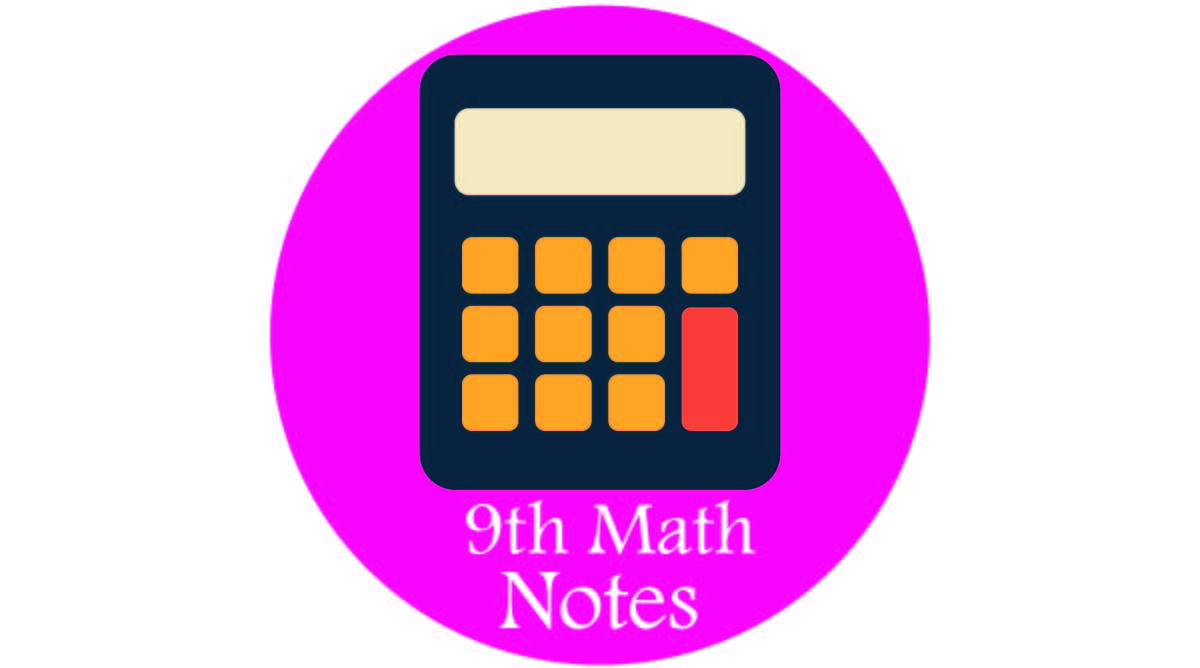# 9th Class Math Chapter Wise

0
219th Class Math Chapter Wise Short or Long Questions and MCQs Notes.

These notes are very well designed, which will be of great benefit to you. With our educational materials, you can prepare more than 100 percent of your marks. This educational material has been overseen by experienced teachers of mathematics. There are 13 chapters of mathematics. Be sure to read it very well explored. This plan is a very important mathematics Question for exam preparation. The complete syllabus of the Math book is described below. You must read it.

## 1- 9th Math (Science) MCQs All Chapter

Unit 1: Matrices and Determinants

Introduction, Matrix, Row and Column Matrix, Order of Matrix, Equal Matrices, EX 1.1, Types of Matrices (Row Matrices, Column Matrix, Rectangular Matrix, Square Matrix, Null or Zero Matrix, Transport of Matrix, Negative of Matrix, Symmetric Matrix, Skew Symmetric, Diagonal Matrix, Scalar Matrix, Identity Matrix) EX 1.2, Addition and Subtraction of Matrices, Multiplication of a Matrix by a Real Numbers.

## 2- 9th Math (Science) Definitions

Unit 2: Real and Complex Numbers

Introduction, Real Numbers ( Natural Numbers, Whole Numbers, Integers), Set of Real Numbers ( Rational Numbers, Irrational Numbers), Depiction of Real Number on Number line, Demonstration of a number with terminating and non-terminating decimals on the number line ( Rational Numbers, terminating decimal fractions, recurring and non-terminating decimal fractions,

## 3- 9th Extra Definition

Unit 3: Logarithms

Introduction, Scientific Notation, EX# 3.1, Logarithm (Logarithm of Real Numbers, Common Logarithm, Characteristics and Mantissa), Using tables to find log of numbers, Concept of Anti-Logarithm and use of Anti-Log tables), EX# 3.2, Laws of Logarithms’ EX# 3.3, Application of Laws of Logarithms in Numerical Calculation, EX # 3.4, Review EX#3.

## 4- 9th Math Short Questions All Chapter

Unit 4: Algebraic Expressions and Algebraic Formulas

Algebraic Expression (Polynomials, Rational Expressional behave like rational numbers), Rational Expression, Properties of Rational Expression, Rational Expression in its Lowest form, Sum, Difference and Product of Rational Expressions, Dividing a Rational expression by another Rational expression,Unit 5: Factorization (Page 97 – 106 )

Introduction, Remainder Theorem and Factor Theorem, To find remainder(without dividing) when a polynomial is divided by a linear polynomial  zero of a polynomial, Ex 5.3 ,Factorization of a cubic polynomial , Rational root Theorem, Ex 5.4, Review Ex# 5

Unit 6: Algebraic Manipulation

Introduction, Highest Common Factor (HCF by factorization and division method) and Least Common Multiple (LCM by factorization) of algebraic expressions, Relation between HCF and LCM, Application of HCF and LCM, EX# 6.1. Square root of Algebraic Expression (Definition by factorization and division), EX# 6.3, Review EX# 6.

Unit 7: Linear Graph and Inequalities

Introduction Linear equations, definitions, solving a linear equation in one variable, Technique for solving, Equations involving radicals but reducible to linear form, EX# 7.1, Equation involving absolute value (Definition, Sum properties of absolute value), Solving linear equations involving absolute value, EX# 7.2 Linear Inequalities, Properties of inequalities (Law of Trichotomy, Transitive Property, Additive Closure Property, Multiplicative Property), Solving linear inequalities, EX# 7.3, Review EX # 7.

Unit 8: Linear Graph and Inequalities

Cartesian Plan and Linear Graphs, An ordered pair of Real Numbers, Recognizing and ordered pair, Cartesian plan, identification of Region and Coordinate axes, Location of point in plan, Drawing different geometrical shapes in Cartesian plan (Line Segment, Triangle, Rectangle), Construction of table for pair of values satisfying linear equation in two variables, plotting the points to get the graph, Scale of Graph, Drawing Graphs of the following Equations, Drawing Graph from a given table of Discrete Values, Conversion Graphs, Reading a given Graph, EX# 8.2,  8.3, Review Exercise 8.

Unit 9: Introduction to Coordinate Geometry

Distance Formula, EX# 9.1, Collinear and Non-collinear points, Use of Distance formula for different shapes, EX# 9.2, Midpoint formula and its verification, EX# 9.3, Review EX# 9.

Unit 10: Congruent Triangles

Congruency of  Triangles, Theorem #10.1.1, EX# 10.1, Theorem # 10.1.2, EX# 10.2, Theorem# 10.1.3, EX# 10.3, Theorem# 10.1.4, EX# 10.4, Review# 10.

Unit 11: Parallelograms and Triangles (Page 199 – 208 )

Theorem 11.1.1, 11.1.2, 11.1.3, 11.1.4, 11.1,5, with corollaries, Ex. 11.1, 11.2,  11.3,  11.4,  11.5,  Review Ex. 11

Unit 12: Line bisectors and angle bisectors (Page 210 – 217)

Introduction, Right bisector of a line segment and Bisector of an angle, Theorem 12.1.1, 12.1.2, 12.1.3, 12.1.4, 12.1.5, 12.1.6, Ex12.1, 12.2, 12.3, Review EX# 12.

Unit 13: Side and Angles of a Triangle (Page 218 – 227)

Theorem 13.1.1, 13.1.2, 13.1.3, 13.1.4, and Corollaries, EX# 13.1, 13.2, Review EX# 13.

Unit 14: Ration and Proportion (Page 228 – 237)

Theorem 14.1.1, 14.1.2, 14.1.3, 14.1.4 and Corollaries, EX# 14.1, 14.2, Review EX# 14.

Unit 15: Pythagoras Theorem (Page 238 – 243)

Theorem# 15.1.1, 15.1.2, EX# 15, Review EX# 15.

Introduction, Some Preliminaries (Area of Triangular Region, Congruent Area axiom, Rectangular Region between the same parallels,

Unit 16: Pythagoras Theorem (Page 244 – 248)

Theorem# 16.1.1, 16.1.2 EX# 16.1 Theorem# 16.1.1, 16.1.2, EX# 16.2, Review EX# 16.EX# 17.1

Unit 17: Practical Geometry (Page 256 – 261) Drawing angle bisector, Altitude, Perpendicular bisector and medians of given triangles, EX# 17.2. Figures with Equal Areas EX# 17.3, 17.4, 17.5, Review EX# 17. Figures with Equal Areas EX# 17.3, 17.4, 17.5, Review EX# 17.

This site uses Akismet to reduce spam. Learn how your comment data is processed.